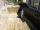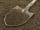# Cuboid + body volume - math problems

A cuboid is a three-dimensional shape with a length, width, and a height. A cuboid is a rectangular Prism. The cuboid shape has six sides called faces. Each face of a cuboid is a rectangle, and all of a cuboid's corners (called vertices) are 90-degree angles. Opposite faces are parallel. A cuboid has the shape of a rectangular box.

1. The tankThe tank is full up to 4/5 of the total height and contains 240 hl of water. The area of the base is 6 square meters. What is the height of the tank?
2. Solid cuboidA solid cuboid has a volume of 40 cm3. The cuboid has a total surface area of 100 cm squared. One edge of the cuboid has length 2 cm. Find the length of a diagonal of the cuboid. Give your answer correct to 3 sig. Fig.
3. Base of houseCalculate the volume of the bases of a square house, if the base depth is 1.2 m, the width is 40 cm and their outer circumference is 40.7 m.
4. Cube, cuboid, and sphereVolumes of a cube and a cuboid are in ratio 3: 2. Volumes of sphere and cuboid are in ratio 1: 3. In what ratio are the volumes of cube, cuboid, and sphere?
5. BasenHow many square meters of tiles we need to tile the walls and floor of the pool 15 meters long, six meters wide and two meters?deep
6. Cubes into cuboidHow many 12 centimeter cubes fit into the block (cuboid) with 6dm, 8,4dm and 4,8?
7. RainIt rains at night. On 1 m2 of lake will drop 60 liters of water. How many cm will the lake level rise?
8. A residentialA residential colony has a population of 5400 and 60 litres of water is required per person per day. For the effective utilization of rain water, they constructed a water reservoir measuring 48m × 27m × 25m to collect the rain water. For how many days, th
9. How muchHow much money will we pay for 20 planks 4m long, 15cm wide and 26mm thick when 1m³ of wood costs 4500kč?
10. Surface of cubesPeter molded a cuboid 2 cm, 4cm, 9cm of plasticine. Then the plasticine split into two parts in a ratio 1:8. From each part made a cube. In what ratio are the surfaces of these cubes?
11. Annual rainfallThe average annual rainfall is 686 mm. How many liters will fall on the 1-hectare field?
12. Water tankA 288 hectoliter of water was poured into the tank with dimensions 12 m and 6 m bottom and 2 m depth. What part of the volume of the tank water occupied? Calculate the surface of tank wetted with water.
13. Water blockA block with a 50 cm2 base is filled with water 5 cm under the edge. How many can sugar cubes with 2 cm edge be thrown into a container that overflow water?
14. Volume and body diagonalCalculate how much the volume and body diagonal of the cuboid decrease if we reduce each of its three edges a, b, c by 18%?
15. RainfallA rectangular garden of 25m in length and width 20m in width fall 4mm of water. Express by a fraction in basic form what part of the 60-hectolitre tank we would fill with this water.
16. ExcavationExcavation for the base of the cottage 4.5 m x 3.24 m x 60 cm. The excavated soil will increase its volume by one-quarter. Calculate the volume of excavated soil.
17. Two cuboidsFind the volume of cuboidal box whose one edge is: a) 1.4m and b) 2.1dm
18. ClayHow many cubic centimeters of clay is in a pit of dimensions 4 m x 3 m x 3 m?
19. TrenchThe trench is a four-sided prism. The cross section has a trapezoidal shape with basements of 4m and 6m, the length of the trench is 30m. What is the depth of the trench if we dig 60,000 l of soil.
20. CuboidCuboid has a surface of 516 cm2. Side a = 6 cm and b = 12 cm. How long is the side c =?

Do you have an interesting mathematical word problem that you can't solve it? Enter it, and we can try to solve it.

We will send a solution to your e-mail address. Solved examples are also published here. Please enter the e-mail correctly and check whether you don't have a full mailbox.

Please do not submit problems from current active competitions such as Mathematical Olympiad, correspondence seminars etc...